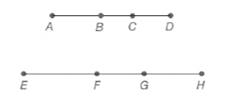Chapter 5.6, Problem 3E### Elementary Geometry for College St...

6th Edition
Daniel C. Alexander + 1 other
ISBN: 9781285195698

#### Solutions

Chapter
Section### Elementary Geometry for College St...

6th Edition
Daniel C. Alexander + 1 other
ISBN: 9781285195698
Textbook Problem
3 views

# Given that A B E F = B C F G = C D G H , are the following proportions true?a) A C E G = C D G H b) A B E F = B D F H

To determine

a)

To find:

Whether the proportion ACEG=CDGH is true or not.

Explanation

Given:

Given that ABEF=BCFG=CDGH for the line segments shown below.

Property used:

If ab=cd then a+cb+d=ab=cd.

Calculation:

Given: ABEF=BCFG=CDGH

Consider ABEF=B

To determine

b)

To find:

Whether the proportion ABEF=BDFH is true or not.

### Still sussing out bartleby?

Check out a sample textbook solution.

See a sample solution

#### The Solution to Your Study Problems

Bartleby provides explanations to thousands of textbook problems written by our experts, many with advanced degrees!

Get Started

#### Simplify the expressions in Exercises 97106. 21/32122/321/3

Finite Mathematics and Applied Calculus (MindTap Course List)

#### Explain what is meant by the statement limx2f(x)=3.

Applied Calculus for the Managerial, Life, and Social Sciences: A Brief Approach

#### 2. Define the terms population, sample, parameter and statistic.

Statistics for The Behavioral Sciences (MindTap Course List)

#### Evaluate the integrals in Problems 1-32. 14.

Mathematical Applications for the Management, Life, and Social Sciences

#### For f(x)=exx,f(x)= a) ex b) xex1x2 c) exxexx2 d) xexxx2

Study Guide for Stewart's Single Variable Calculus: Early Transcendentals, 8th

#### True or False:

Study Guide for Stewart's Multivariable Calculus, 8th